# Implementing K-means Clustering Algorithm with Python

## Introduction to k-means Clustering Algorithm

If you compare supervised learning and unsupervised learning in terms of understanding, supervised seems to be an easy one but, after going through K means clustering algorithm, your perception towards this comparison could change. K means clustering is an unsupervised algorithm which means that we don’t have any labels associated with our machine learning dataset. This unsupervised machine learning algorithm is used to find the patterns among the unlabelled dataset to label them.

## How does Clustering work?

The first step towards understanding k means clustering is to understand what clustering means, clustering is a method where similar data-points in a dataset can be grouped together, in this way we would have each cluster with a unique feature or pattern. Consider a group of mango and apple images is fed to the K means algorithm, what we shall get is, a separate image group of mangoes and a separate group of apple images.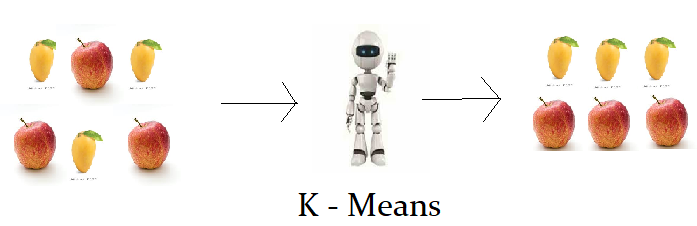With the above example, we can figure out that K means algorithm is recognizing patterns on similar data and giving clusters of those similar patterns as an output. In machine learning, these clusters are used in many applications and are very valuable data resources.

## Types of Clustering in K means Clustering

Clustering depends upon the algorithm to algorithm, and we can see different types of clustering using the same datasets on the different algorithms. Clustering is of three types:-

### Exclusive Clustering

Just as in a Walmart, Bananas are not stacked up with mangoes, even though they belong to the group of fruits. Similarly, whenever data is existing only in one group or cluster exclusively, it is known as exclusive clustering. Example -> Kmeans clustering.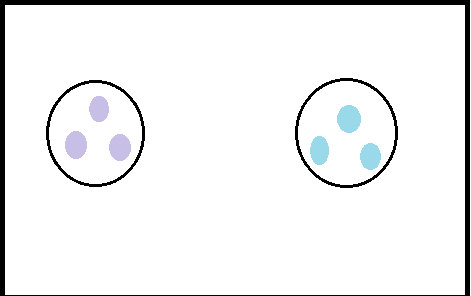### Overlapping Clustering

when some data points from one cluster show the attributes of another cluster too, it can coincide between the two thus creating an overlapping, this is known as overlapping clustering. Exam - Fuzzy algorithm.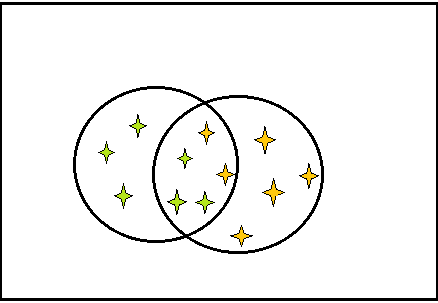### Hierarchical Clustering

Whenever in a single cluster, there are multiple clusters, we could say that the clustering over there is hierarchical clustering. Let us consider A and B have some similarities and D and F have some similarities among them, whereas a combination of A and B is similar to C, and a combination of D and F is similar to E. And at last, the combination of A, B, C is similar to D, E, and F.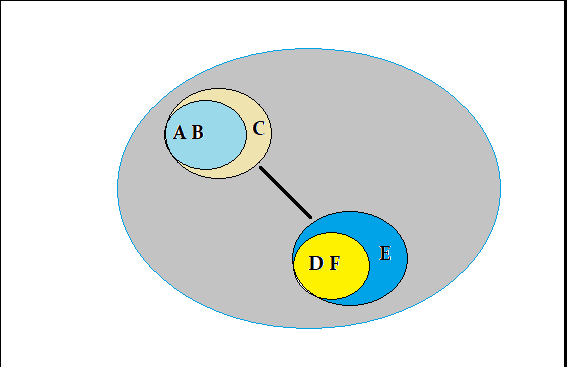## Working Of K-means Clustering

Step 1 -: We will start by determining the number of groups or clusters we need, remember we can always change the number of clusters in order to increase the accuracy of the algorithm, so in K-means clustering we generally denote the number of clusters as K, so if we want to have 4 clusters we will determine the value of K as K=4.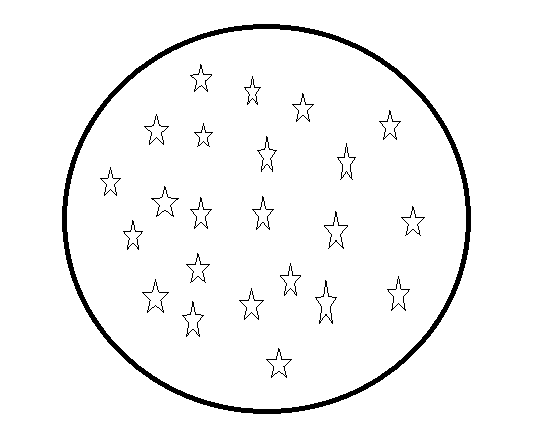Step 2 -: Randomly select a few data points, these data points are in sync with the value of K, i.e if the value of K is 3 then you should select 3 random points as they will act as a centroid for the clusters, the easiest way to generate random data point is to use Numpy library of python.Step 3 -: Our third step is to find out the distance between each random data point we selected to all other data points, so the data points that are nearest to each random point are considered to be the data point belonging to that cluster.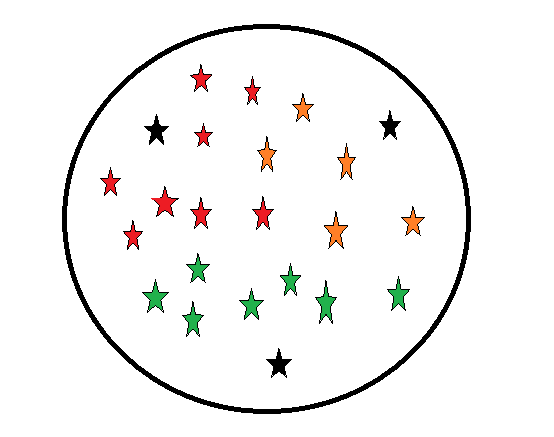## Application of K-means Algorithm in Real World

Some of the applications of K means clustering is -:

1. Document Clustering
2. Hidden Pattern Recognition
3. Image Segmentation
4. Recommendation System
5. Wireless Sensor Network

## Code for K means clustering algorithm Python

``````import numpy as np
import matplotlib.pyplot as plt

#Generating Datasets

mean_01 = [0, 1]
cov_01  = [[2.3, 0.7], [0.7, 1.5]]

mean_02 = [4,3]
cov_02  = [[1.2, -0.5], [-0.5, 1]]

dist_01 = np.random.multivariate_normal(mean_01, cov_01, 500)
dist_02 = np.random.multivariate_normal(mean_02, cov_02, 500)

plt.figure()
plt.scatter(dist_01[:, 0], dist_01[:, 1], color='red')
plt.scatter(dist_02[:, 0], dist_02[:, 1], color='green')
plt.show()``````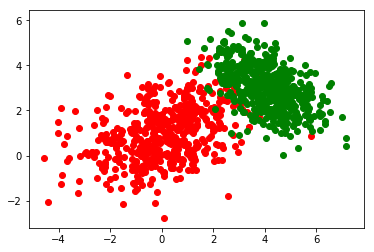Initializing Clusters

``````dataset = np.zeros((dist_01.shape + dist_02.shape, dist_01.shape))

dataset[:dist_01.shape, :] = dist_01
dataset[dist_01.shape:, :] = dist_02

class Cluster:
#     count = 0

def __init__(self, center, color):
self.center = center
self.color = color
self.points = list()
#         self.id = count
#         Cluster.count += 1

def __str__(self):
return "Cluster <{}>".format(self.color)

def __repr__(self):
return "Cluster <{}>".format(self.color)

def update_center(self):
if not self.points:
return self.center

new_center = np.array(self.points).mean(axis=0)
self.center = new_center
return new_center

``````

## Initializing K-means Clustering Algorithm

``````class KMeansClustering:

def __init__(self, k, clusters, dataset, centers):
self.k = k
self.clusters = clusters
self.dataset = dataset
self.centers = centers
self.inertias = list()

def run(self, iterations=1):
for _ in range(iterations):
#             self.calculate_distances()
#             self.assign_cluster()
self.assign_closest_cluster()
self.update_cluster_centers()
self.inertias.append(self.calculate_inertia())

def assign_closest_cluster(self):
for cluster in self.clusters:
cluster.points = list()

for point in self.dataset:

distances = []

for cluster in self.clusters:
d = KMeansClustering.euclidean(point, cluster.center)
distances.append((d, cluster))

distances = sorted(distances, key=lambda x: x)
closest_cluster = distances
closest_cluster.points.append(point)

def update_cluster_centers(self):
new_centers = []
for cluster in self.clusters:
#             new_center = np.array(cluster.points).mean(axis=0)
#             cluster.center = new_center
new_centers.append(cluster.update_center())
self.centers = new_centers

def calculate_inertia(self):
inertia = 0

for cluster in self.clusters:
for point in cluster.points:
inertia += KMeansClustering.euclidean(point, cluster.center)
return inertia

@staticmethod
def euclidean(p1, p2):
return np.sqrt(np.sum((p1 - p2)**2))``````

## Initializing K and plotting clusters

``````k = 5
random_centers = np.random.uniform(low=dataset.min(), high=dataset.max(), size=(k, dataset.shape))
colors = ['red', 'green', 'blue', 'yellow', 'pink']

clusters = []
# for i in range(k):
#     clusters.append(Cluster(random_centers[i], colors[i]))
for center, color in zip(random_centers, colors):
clusters.append(Cluster(center, color))

kmeans = KMeansClustering(k, clusters, dataset, random_centers)

plt.figure()
plt.title("Initial State")
plt.scatter(kmeans.dataset[:, 0], kmeans.dataset[:, 1])
plt.scatter(kmeans.centers[:, 0], kmeans.centers[:, 1], color='black', marker='^', s=100)
plt.show()``````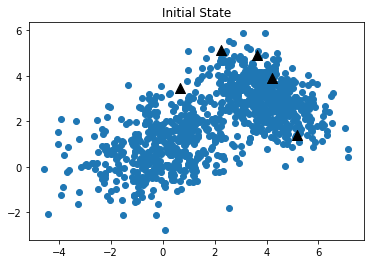## Iterating to find accurate clusters

``````def plot_iteratively(kmeans, iterations=1, inp=True):
for i in range(iterations):
kmeans.run()
plt.figure()
plt.title("Iteration {}".format(i))
for cluster in kmeans.clusters:
points = np.array(cluster.points)
plt.scatter(cluster.center, cluster.center, color='black', marker='^', s=100)
if not cluster.points:
continue
plt.scatter(points[:, 0], points[:, 1], color=cluster.color)

plt.show()
if inp:
input()

inertias = []
plot_iteratively(kmeans, 10, False)``````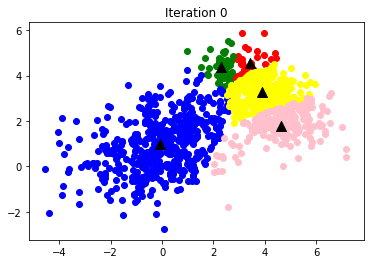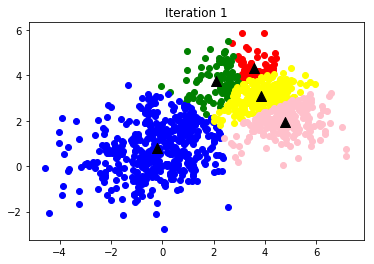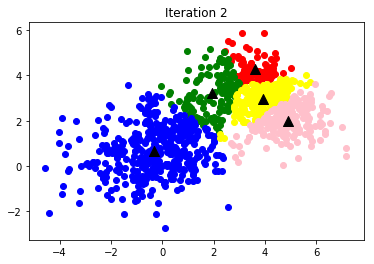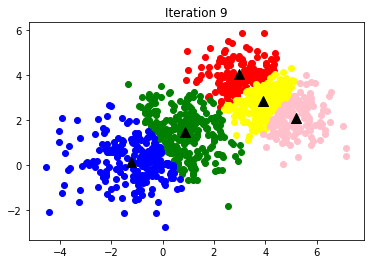### tanesh

Founder Of Aipoint, A very creative machine learning researcher that loves playing with the data.